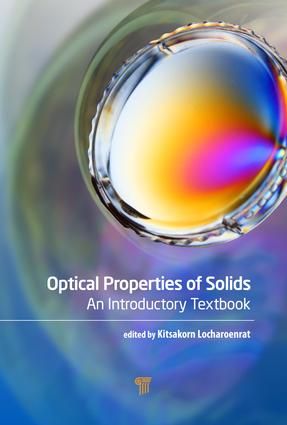Optical Properties of Solids

An Introductory Textbook, 1st Edition

Jenny Stanford Publishing

320 pages | 18 Color Illus. | 182 B/W Illus.

Purchasing Options:\$ = USD
Hardback: 9789814669061
pub: 2016-02-19
SAVE ~\$21.00
\$105.00
\$84.00
x
eBook (VitalSource) : 9780429083440
pub: 2016-03-23
from \$52.50

FREE Standard Shipping!

Description

This textbook presents the general point of views of the optical properties of solids and gives an overview of the landscape of optics in solid-state materials, especially focusing on optical imaging techniques. It presents the background of electromagnetic theory, which is based on Maxwell’s equations. It shows how to manipulate Maxwell’s equations in differential forms by utilizing vector analysis and how to calculate the electric field emerging from a single charge and from charge distributions in conductors and dielectrics under Maxwell’s boundary conditions. It analyzes the optical spectra from localized electronic states and goes over some well-known phenomena currently under research, such as nonlinear optical response of materials. It also gives a background on optical microscopy, focusing on the optical response of modern confocal microscopy on asymmetric materials, and introduces optical tomographic techniques to identify the locations and profiles of matter, concentrating on fluorescence diffuse optical tomography used as a probe in deep biological tissue.

The book is designed for all kinds of learners, especially independent learners, and is aimed to facilitate the visualization of related theoretical concepts. Problem sets have been provided with each chapter to examine the readers’ understanding of each concept.

Reviews

"This is a good book on optical properties of solids. It starts with several introductory chapters on classical and quantum mechanical treatment of light–matter interaction and fundamental optics of solids such as light scattering, light absorption, emission, and lasing. The microscopy is treated in detail, including tunneling, atomic force, electron, and confocal microscopy. The final chapters are focused on optical tomography. I liked the way these subjects are covered and the materials included."

—Prof. Nasser Peyghambarian, University of Arizona, USA

"This book is well organized and can be of great benefit for teaching the subject of optical properties of solid materials to undergraduate students. The text can also be used as handy reference material for researchers that need more understanding on optical properties."

Prof. Pieter Stroeve, University of California, Davis, USA

Preface

Vector Analysis and Maxwell’s Equations

INTRODUCTION

SCALARS AND VECTORS

SCALAR AND VECTOR PRODUCTS

GRADIENT, DIVERGENCE AND ROTATION OF VECTORS

GAUSS’ S AND STOKES’S THEOREM

MAXWELL’S EQUATIONS

PROBLEMS

Electromagnetic Units and Electric Charges

INTRODUCTION

MKS UNITS

COULOMB’S LAW

CONDUCTOR AND INSULATOR

PROBLEMS

Electric Field and Electric Potential

INTRODUCTION

ELECTRIC FIELD

THE LINE OF ELECTRIC FORCE

ELECTRIC POTENTIAL

GAUSS’S LAW

OHM’S LAW

ELECTRIC DIPOLE

PROBLEMS

Capacitance and Electromagnetic Energy

INTRODUCTION

CAPACITANCE

ELECTROMAGNETIC ENERGY

COEFFICIENT OF ELECTROSTATIC POTENTIAL

PROBLEMS

Dielectric Materials

INTRODUCTION

POLARIZATION

ELECTRIC DISPLACEMENT AND DIELECTRIC CONSTANT

MAXWELL’S BOUNDARY CONDITIONS

PROBLEMS

Methods of Determining Electric Field and Potential

INTRODUCTION

LAPLACE-POISSON EQUATION

METHOD OF IMAGES

DIRECT SOLUTION

CONFOCAL MAPPING

GREEN’ S FUNCTION

PROBLEMS

Light

INTRODUCTION

LIGHT

DE BROGLIE WAVES

CABLE WAVES

ELECTROMAGNETIC WAVES

PROBLEMS

Classical and Quantum Theory of Light

INTRODUCTION

ELECTROMAGNETIC WAVES IN VACUUM

POLARIZATION OF LIGHT

SPECTRUM OF LIGHT

ELECTROMAGNETIC WAVES IN MEDIUM

ELECTROMAGNETIC POTENTIAL

ELECTROMAGNETIC FIELD QUANTIZATION

PROBLEMS

Classical Theory of Light-Matter Interaction I

INTRODUCTION

OPTICAL CONSTANTS

PROPAGATION OF LIGHT IN A MATERIAL

KRAMERS-KRONIG RELATIONS

PROBLEMS

Classical Theory of Light-Matter Interaction II

INTRODUCTION

LORENTZ MODEL

DRUDE MODEL

THEORY OF LOCAL FIELD

PROBLEMS

Quantum Theory of Light-Matter Interaction

INTRODUCTION

QUANTUM THEORY OF MATTER

SEMI-CLASSICAL QUANTUM MECHANICAL TREATMENT

FULLY QUANTUM MECHANICAL TREATMENT

PROBLEMS

Electron-Nuclei Interaction

INTRODUCTION

SEPARATION OF MOTIONS OF ELECTRONS AND NUCLEI

MOLECULAR VIBRATIONS

ABSORPTION SPECTRUM

ORIGIN OF SPECTRAL PROFILE

PROBLEMS

Optical Spectra of Materials

INTRODUCTION

OPTICAL SPECTRA OF ATOMS

OPTICAL SPECTRA OF MOLECULES

OPTICAL SPECTRA OF CRYSTALS

PROBLEMS

Some Interesting Phenomena

INTRODUCTION

LUMINESCECE

LIGHT SCATTERING

LASER ACTION

OPTICAL SECOND HARMONIC GENERATION

PROBLEMS

Optics of Eyes

INTRODUCTION

COMPONENT OF EYES

FUNCTION OF EYES

OCULAR PROBLEM AND ITS CORRECTION

PROBLEMS

Appendix A

Index